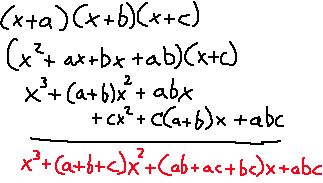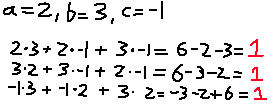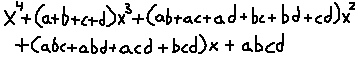# Commutative Operations from Binomials

Ever notice that when expanding two binomial factors that you can figure out two of the terms by adding and multiplying the numbers together? Here's an example.

``` (x + 3) (x + 2) ```

If you want to expand this, you know it will be x^2 for the first part. What about the x and constant term? For the x term, that will just involve summing the 3 and the 2, making 5. For the constant, you multiply the 3 and the 2 together to get 6. Putting it all together, you get:

``` x^2 + 5x + 6 ```

This will work with any two binomial factors! But, what happens when you put a third one into them mix? Things are not so obvious.

```            ```
(x + 3) (x + 2) (x - 1)
(x^2 + 5x + 6) (x - 1)
x^3 + 4x^2 + x - 6
```
```

Here, the numbers to work with are 2, 3, and -1. If you add them all together, you end up with 4, and that happens to match up with the x^2 term! Multiplying 2, 3, and -1, you get -6, which is the same as the constant term here! Then where does this "x" term come from? Is there some simple operation to calculate that one too?

This will be easier to figure out if we generalize the numbers into variables and perform the expansion again.That's a bit more complicated than expected, but there it is. ab + ac + bc. It's basically taking every 2-combination of a, b, c (order doesn't matter), and adding them all together.

What's really cool is that ab + ac + bc as a whole is a commutative operation, just like addition and multiplication! What that means is that you can swap the variables around any way you want, and you will end up with the same result. Here's an example:If it even makes remote sense, as the power of each term decreases, the values of the terms use less addition and more multiplication. For x^2, it's adding every constant. For x, it's multiplying pairs of constants but adding them together. For the constant term, it's all of them multiplied.

What does this look like for when we have four binomial factors?Wait a second. I see a pattern. First of all, the number of constants in each term is 1,4,6,4,1. Of course one of the rows of Pascal's Triangle shows up here. That also means that combinations are involved. In the x^3 term, every 1-combination of constants are used and summed together. In the x^2 term every 2-combination of constants are used and summed together. In the x term every 3-combination of constants are used and summed together. For the constant, there is only one combination involving four terms, so abcd is the only value there. Every one of those values for the terms is a commutative operation for four values!

Is this easier to remember for how to factor out large binomial factors? Yeah, I say so. I can type out the expansion for 6 terms off the top of my head now: